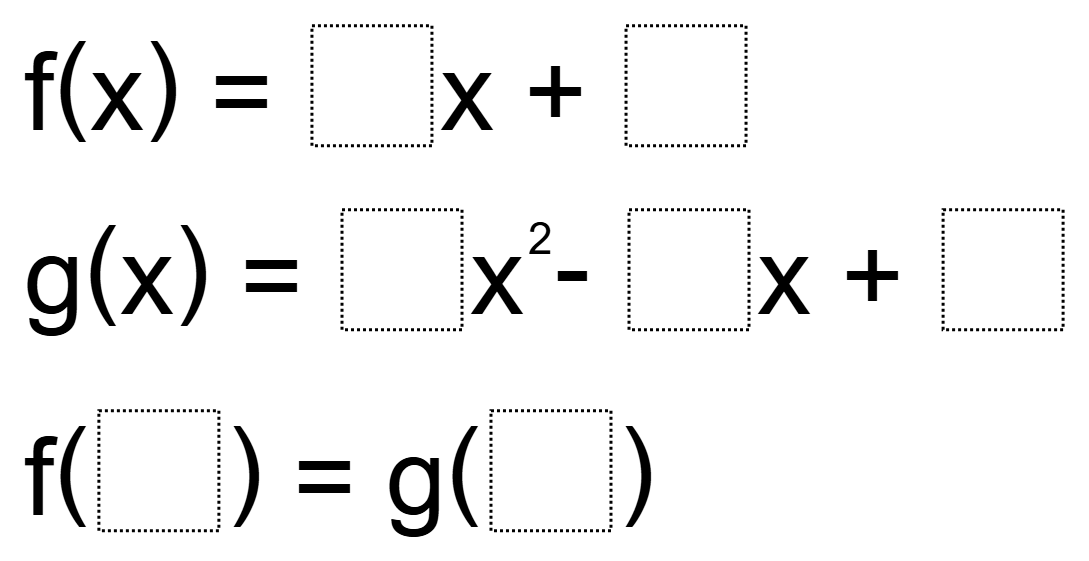# Function Notation

Directions: Using the digits 1 to 9, at most one time each, fill in the boxes so that the values of the two functions are equivalent### Hint

How do you use function notation to determine the input and output of a function?

One possible answer is:
f(x) = 9x + 4
g(x) = 7x^2 – 1x + 5
f(3) = g(2)

Post any others in the comments.

Source: Steven Midzak

## Asymptotes of Rational Functions

Directions: Using the digits 1 to 9 at most one time each, place a digit …

1.I think the format is confusing, and the answer uses incorrect notation and the two functions are, in fact, never “equivalent”. Rephrase like this?

Fill in spaces with whole numbers 1 – 9 only one time, so that the final equality is correct.
f(x) = ___ x + _____
g(x) = ___ x^2 – ____x + _____
f(____) = g(_____)

•I agree. I like the format you used better Tim.

•Thank you for this suggestion. I’ve edited the question accordingly.

•It doesn’t appear to be edited.

2.f(3) = 2x + 4
g(1) = 7x^2 – 6x + 9

3.F(3)=9x+4 g(2)=7x^2-1x+5

4.5.Katy Howell Escobar

𝑓(3)=7𝑥+6 𝑔(2)=5𝑥^2−1𝑥+9

𝑓(8)=6𝑥+4 𝑔(5)=2𝑥^2−1𝑥+7

6.I gave this to my IM3 classes today, here are some they came up with that are NOT listed above:

f(3) = 6x + 1 g(4) = 2x^2 – 5x + 7
f(8) = 2x + 3 g(6) = 1x^2 – 4x + 7
f(1) = 3x + 6 g(2) = 4x^2 – 8x + 9
f(2) = 3x + 4 g(1) = 7x^2 – 5x + 8
f(2) = 3x + 7 g(5) = 1x^2 – 4x + 8

•May I ask how you got your solutions

7.I should have looked at the solutions earlier. That negative before the x term in the quadratic makes all the difference as per Tim’s comment waaaaay back. I wonder why the question as posted still shows the +.

Thank you for this site, it is awesome!

•While the negative sign does make it easier, it is possible to solve with the + in g(x) as originally listed.

f(x) = 7x + 2; g(x)=1x^2 + 3x + 4
f(6) = g(5) = 44

8.f(x) = 8x + 5; g(x) = 3x^2-x+9
f(6) = g(4) = 53

9.Love the problem and this site. Thanks.

I gave this to my 8th graders today and one of the students took off with it and ran. He wrote a program that generated 988 possible answers. And that’s why open middle questions can be so much fun. So many ways to do this yet it still stumped a lot of my students at first.

•Wow! I’m curious, are all the 988 possibilities within the constraints of the problem (no repeating digits)?

•There are 36 ways to pick 7 digits out of 9, and there are 7! ways to arrange each set of 7 digits, so (36)(7!)=181,440 total possible arrangements that do not repeat a number.